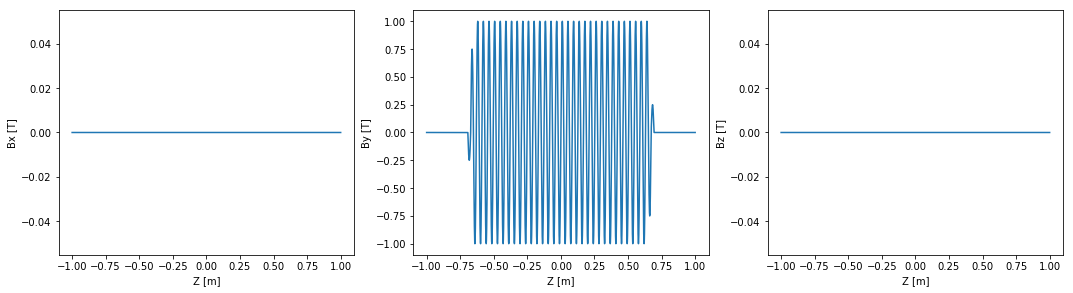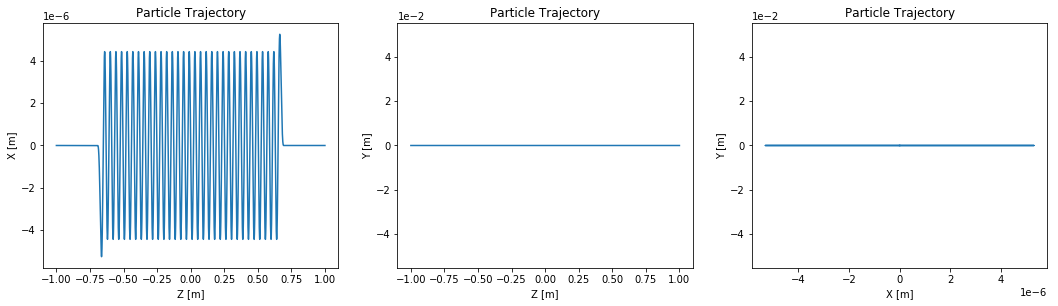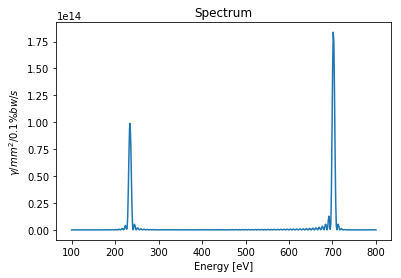This document briefly explains how the GPU and multi-threading is used in OSCARS.

The simplest way to use threads and the GPU is to specify it when creating an oscars.sr object:

# Create a new OSCARS object.  Default to 8 threads and always use the GPU if available



This will set the defaults to use 8 threads (choose however many you like) and to use as many GPUs as are available. The GPU has precidence over multi-threading. Nothing else is needed unless you wish to use the GPU/threads on individual function calls, then see below.

Nearly all functions in oscars.sr accept the argument 'gpu=1' and 'gpu=0'. If you select 1, oscars will use the gpu to do the calculation. All of these functions also accept the argument 'ngpu=X' where X is the number of GPUs you which to use as well as 'ngpu=[n0, n1, ...]' where n0, n1, ... are the gpu numeric identifiers of the GPUs you wish to use. You may find these using the command:

osr.print_gpu()



Nearly all functions in oscars.sr accept an argument like 'nthreads=123' where 123 is the number of threads you wish to use for a calculation.

At the moment the GPU has higher precident than threads. This means that if you attempt to use both, the gpu will be enabled without multi-threading.

You MAY use the GPU or multi-threading with MPI, however the user should take care that the distribution of resources makes sense.

You can also set the gpu and nthreads global flags inline anywhere if you like:

osr.set_gpu_global(1)



You can check if you have a gpu that oscars can see. This will return the number of GPUs that oscars can see, or -1 if your version was not compiled with GPU support.

osr.check_gpu()



## When is the GPU or multi-threading useful?¶

Always. Even if you are calculating a single-particle spectrum, the points in the spectrum are handed to different threads (on the CPU or GPU). If you are looking at a 2D or 3D flux or power density the different points are distributed. There is some overhead in copying data over to the GPU, but this is almost always outweighed by the GPU performance as compared to a typical workstation.

## Problems running on the GPU?¶

In order for OSCARS to use your GPU the driver must be correctly installed for your operating system. At the moment it also must be an nvidia cuda-compatible card (quite common).

In :
# matplotlib plots inline
%matplotlib inline

# Import the OSCARS SR module
import oscars.sr

# Import OSCARS plots (matplotlib)
from oscars.plots_mpl import *

OSCARS v2.1.8 - Open Source Code for Advanced Radiation Simulation
Brookhaven National Laboratory, Upton NY, USA
http://oscars.bnl.gov
oscars@bnl.gov

In :
# Create a new OSCARS object.  Default to 8 threads and always use the GPU if available

In :
# Will return the number of GPUs available, or -1 and print an error
# If you built OSCARS yourself with setup.py it will likely not have GPU support
# builtin.  The binary versions available for download all have this builtin

osr.check_gpu()

Out:
1
In :
# Print gpu information
osr.print_gpu()

*GPUs*
Use GPU Globally: 1
Number of GPUs: 1

GPU 0
Memory Clock Rate (KHz): 2700000
Memory Bus Width (bits): 256
Peak Memory Bandwidth (GB/s): 172.800000


In :
# For these examples we will make use of a simple undulator field
osr.add_bfield_undulator(bfield=[0, 1, 0], period=[0, 0, 0.042], nperiods=31)

# Plot the field
plot_bfield(osr)## Beam¶

Add a basic beam somewhat like NSLS2. All that is below also works for multi-particle simulations

In :
# Add a basic electron beam with zero emittance
osr.set_particle_beam(energy_GeV=3, x0=[0, 0, -1], current=0.500)

# You MUST set the start and stop time for the calculation
osr.set_ctstartstop(0, 2)

# Plot trajectory of beam
osr.set_new_particle()
plot_trajectory_position(osr.calculate_trajectory())## Spectrum¶

If you set the global settings for gpu or nthreads you do not need to specify it in each function call, but if you do it will override any global settings.

In :
# Use multi-threading
spectrum = osr.calculate_spectrum(obs=[0, 0, 30], energy_range_eV=[100, 800], npoints=500, nthreads=8)
plot_spectrum(spectrum)In :
# Use the GPU
spectrum = osr.calculate_spectrum(obs=[0, 0, 30], energy_range_eV=[100, 800], npoints=500, gpu=1)
plot_spectrum(spectrum)In :
# Use specific number of GPUs, in the case use 3 GPUs (if available)
spectrum = osr.calculate_spectrum(obs=[0, 0, 30], energy_range_eV=[100, 800], npoints=500, ngpu=3)
plot_spectrum(spectrum)In :
# Use specific GPUs, in the case GPUs numbered 0, 2, and 3
spectrum = osr.calculate_spectrum(obs=[0, 0, 30], energy_range_eV=[100, 800], npoints=500, ngpu=[0, 2, 3])
plot_spectrum(spectrum)## Other Calculations¶

Thread and GPU specifications for flux and power density calculations are exactly the same as above